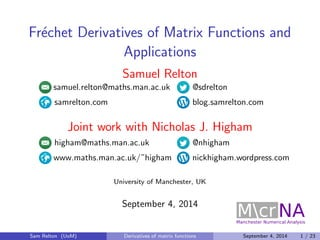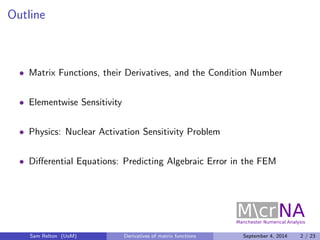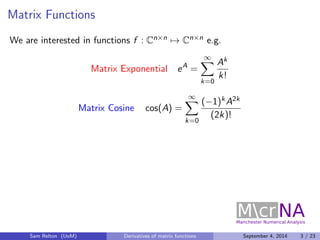Successfully reported this slideshow.

# Frechet Derivatives of Matrix Functions and Applications

2

Share×
1 of 30
1 of 30

# Frechet Derivatives of Matrix Functions and Applications

2

Share

I discuss some recent ideas using the Frechet derivative of matrix functions to analyze the mixed condition number, solve the nuclear activation sensitivity problem, and analyze the distribution of the algebraic error in the finite element method.

Originally presented at the 4th IMA Conference on Numerical Linear Algebra and Optimization, Birmingham, UK. 4th September 2014.

Joint work with Nicholas J. Higham, Wayne Arter, Zdenek Strakos, and Jan Papez.

I discuss some recent ideas using the Frechet derivative of matrix functions to analyze the mixed condition number, solve the nuclear activation sensitivity problem, and analyze the distribution of the algebraic error in the finite element method.

Originally presented at the 4th IMA Conference on Numerical Linear Algebra and Optimization, Birmingham, UK. 4th September 2014.

Joint work with Nicholas J. Higham, Wayne Arter, Zdenek Strakos, and Jan Papez.

## More Related Content

### Related Books

Free with a 14 day trial from Scribd

See all

### Related Audiobooks

Free with a 14 day trial from Scribd

See all

### Frechet Derivatives of Matrix Functions and Applications

1. 1. Frechet Derivatives of Matrix Functions and Applications Samuel Relton samuel.relton@maths.man.ac.uk @sdrelton samrelton.com blog.samrelton.com Joint work with Nicholas J. Higham higham@maths.man.ac.uk @nhigham www.maths.man.ac.uk/~higham nickhigham.wordpress.com University of Manchester, UK September 4, 2014 Sam Relton (UoM) Derivatives of matrix functions September 4, 2014 1 / 23
2. 2. Outline Matrix Functions, their Derivatives, and the Condition Number Elementwise Sensitivity Physics: Nuclear Activation Sensitivity Problem Dierential Equations: Predicting Algebraic Error in the FEM Sam Relton (UoM) Derivatives of matrix functions September 4, 2014 2 / 23
3. 3. Matrix Functions We are interested in functions f : Cnn7! Cnn e.g. Matrix Exponential eA = 1X k=0 Ak k! Matrix Cosine cos(A) = 1X k=0 (